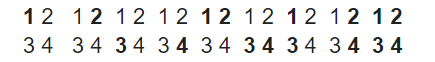New update is available. Click here to update.

# Sub Matrices With Sum Zero

Last Updated: 23 Jan, 2021
Difficulty: Moderate

## PROBLEM STATEMENT

#### NOTE:

``````1. The matrix may also contain some negative elements.
2. A square matrix is a matrix with the same number of rows and columns.
A matrix obtained by deleting some (possibly zero) of the rows and/or columns from the beginning and/or from the end of a matrix is said to be a sub-matrix of the given matrix.

Example: Given a matrix
A = 1 2
3 4

Possible non-empty sub-matrices of A are represented below by bold numbers-
``````##### Input Format:
``````The first line of input contains T, denoting the number of test cases.

The first line of each test case contains an integer N, the order of the square matrix.

The following N lines contain N space-separated integers, representing the elements in the ith row of the matrix 'MAT'.
``````
##### Output Format:
``````The only line of output of each test case should contain an integer denoting the number of non-empty sub-matrices such that the sum of all the elements inside the sub-matrix is equal to zero.
``````
##### Note:
``````You don’t need to print anything. It has already been taken care of. Just implement the given function.
``````
##### Constraints:
``````1 <= T <= 5
1 <= N <= 70

Time Limit: 1 sec
``````## Approach 1

As it is clear from the hint itself, we will start by converting this problem to a 1D version. Let’s say we have a matrix name M. Now to convert M from 2D to 1D, we will try to compress the columns between 2 fixed rows, and then we will solve the 1D version on the compressed array. Let’s understand how we will achieve this.

• Now, we will traverse each row from 1 to N. Now, let's suppose we’re at row 1 and in each row, we will start from a column i.e from 1 to N.
• Now we will run a loop to fix each row from 1 to N(loop variable i) once and repeat the following process for a particular row when it is fixed.
• For example, consider a matrix :

8  5  7

3  7 -8

5 -8  9

• When row i of our submatrix has been fixed (in our example it is -8 5 7). Now we will try to make all the possible submatrices with different numbers of rows with the condition that the first row is fixed i.e Row 1.
• Since we have fixed row 1, let’s try out all the possible submatrices. We do this by running a loop from i till N(loop variable j).
• Case1: Submatrix with a number of rows=1:

-8 5 7

• Case2: Submatrix with a number of rows=2:

-8 5 7

3 7 -8

• Case3: Submatrix with a number of rows=3:

-8 5 7

3 7 -8

5 -8 9

• As we are only concerned with the sum of the submatrices we will try to convert this 2D submatrix array into a 1D array and from this array, we will find the number of subarrays that equals zero.
• We do this by running a third nested loop(loop variable k) from 0 till N.
• In case 1 the subarray will be -8 5 7. In case 2 the subarray will be an addition of row 1 and row 2, hence the subarray becomes -8+3, 5+7, 7-8 = -5 12 -1. In case 3, the subarray will be the addition of all the three rows -8+3+5, 5+7+8, 7-8+9 = 0 20 8
• Now we can see here that a subarray of this 1D array will be representing a particular submatrix. Now our question becomes to find the number of subarrays whose sum equals 0.
• Let’s start by creating a hash table, which we will use to store the sum of the subarrays.
• Now for each 1 D array that we have obtained, let's do the following operations to find the subarrays with a sum equal to 0.
• Maintain the sum of elements encountered so far in a variable (say sum) and count variable initialized to be 0.
• If the current sum is 0, we found a subarray starting from index 0 and ending at index current index, hence we increment our count by 1.
• Check if the current sum exists in the hash table or not.
• If the current sum already exists in the hash table then it indicates that this sum was the sum of some sub-array elements ARR…ARR[i] and now the same sum is obtained for the current sub-array ARR…ARR[j] which means that the sum of the sub-array ARR[i+1]…ARR[j] must be 0.
• Insert current sum into the hash table.
• Our final answer will be the sum of all the counts that we have received from all of the 1D arrays.